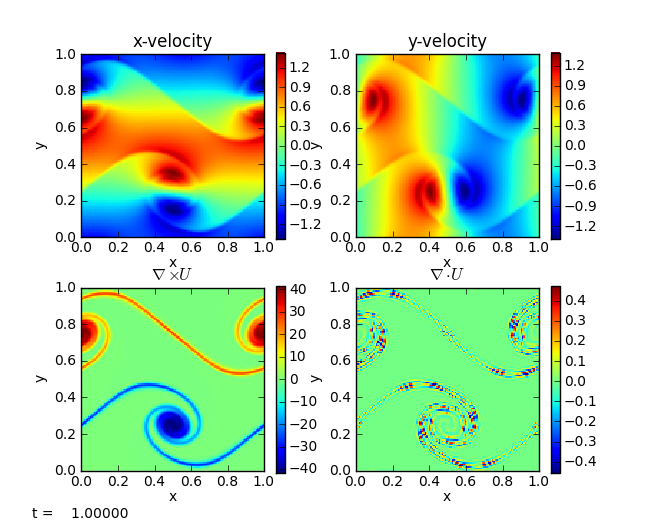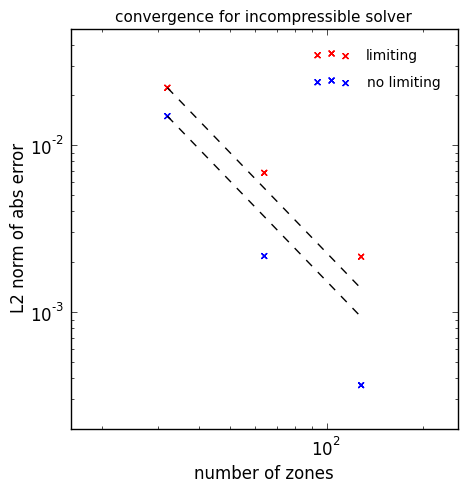# Incompressible hydrodynamics solver

pyro’s incompressible solver solves:

$\begin{split}\frac{\partial U}{\partial t} + U \cdot \nabla U + \nabla p &= 0 \\ \nabla \cdot U &= 0\end{split}$

The algorithm combines the Godunov/advection features used in the advection and compressible solver together with multigrid to enforce the divergence constraint on the velocities.

Here we implement a cell-centered approximate projection method for solving the incompressible equations. At the moment, only periodic BCs are supported.

The main parameters that affect this solver are:

• section: [driver]

option

value

description

cfl

0.8

• section: [incompressible]

option

value

description

limiter

2

limiter (0 = none, 1 = 2nd order, 2 = 4th order)

proj_type

2

what are we projecting? 1 includes -Gp term in U*

• section: [particles]

option

value

description

do_particles

0

particle_generator

grid

## Examples

### shear

The shear problem initializes a shear layer in a domain with doubly-periodic boundaries and looks at the development of two vortices as the shear layer rolls up. This problem was explored in a number of papers, for example, and . This is run as:


span.prompt1:before {
content: "\$ ";
}
pyro_sim.py incompressible shear inputs.shearThe vorticity panel (lower left) is what is usually shown in papers. Note that the velocity divergence is not zero—this is because we are using an approximate projection.

### convergence

The convergence test initializes a simple velocity field on a periodic unit square with known analytic solution. By evolving at a variety of resolutions and comparing to the analytic solution, we can measure the convergence rate of the algorithm. The particular set of initial conditions is from [Minion96]. Limiting can be disabled by adding incompressible.limiter=0 to the run command. The basic set of tests shown below are run as:

pyro_sim.py incompressible converge inputs.converge.32 vis.dovis=0
pyro_sim.py incompressible converge inputs.converge.64 vis.dovis=0
pyro_sim.py incompressible converge inputs.converge.128 vis.dovis=0


The error is measured by comparing with the analytic solution using the routine incomp_converge_error.py in analysis/. To generate the plot below, run

python incompressible/tests/convergence_errors.py convergence_errors.txt


or convergence_errors_no_limiter.txt after running with that option. Then:

python incompressible/tests/convergence_plot.pyThe dashed line is second order convergence. We see almost second order behavior with the limiters enabled and slightly better than second order with no limiting.

## Exercises

### Explorations

• Disable the MAC projection and run the converge problem—is the method still 2nd order?

• Disable all projections—does the solution still even try to preserve $$\nabla \cdot U = 0$$?

• Experiment with what is projected. Try projecting $$U_t$$ to see if that makes a difference.

### Extensions

• Switch the final projection from a cell-centered approximate projection to a nodal projection. This will require writing a new multigrid solver that operates on nodal data.

• Add viscosity to the system. This will require doing 2 parabolic solves (one for each velocity component). These solves will look like the diffusion operation, and will update the provisional velocity field.

• Switch to a variable density system. This will require adding a mass continuity equation that is advected and switching the projections to a variable-coefficient form (since ρ now enters).

## Going further

The incompressible algorithm presented here is a simplified version of the projection methods used in the Maestro low Mach number hydrodynamics code. Maestro can do variable-density incompressible, anelastic, and low Mach number stratified flows in stellar (and terrestrial) environments in close hydrostatic equilibrium.